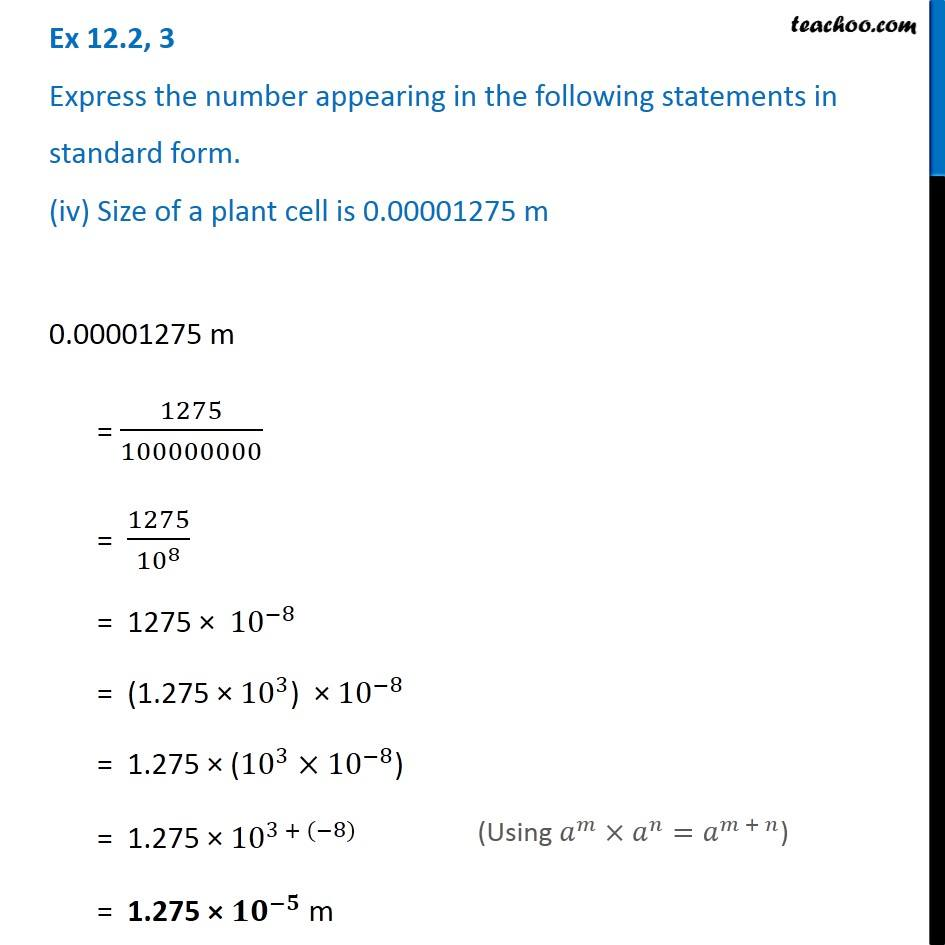Ex 12.2

Chapter 12 Class 8 Exponents and Powers
Serial order wiseIntroducing your new favourite teacher - Teachoo Black, at only ₹83 per month

### Transcript

Ex 12.2, 3 Express the number appearing in the following statements in standard form. (iv) Size of a plant cell is 0.00001275 m 0.00001275 m = 1275/100000000 = 1275/〖10〗^8 = 1275 × 〖10〗^(−8) = (1.275 × 〖10〗^3) × 〖10〗^(−8) = 1.275 × (〖10〗^3×〖10〗^(−8)) = 1.275 × 〖10〗^(3 + (−8)) = 1.275 × 〖𝟏𝟎〗^(−𝟓) m (Using 𝑎^𝑚×𝑎^𝑛=𝑎^(𝑚 + 𝑛))